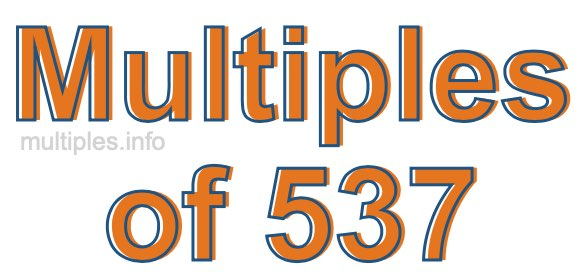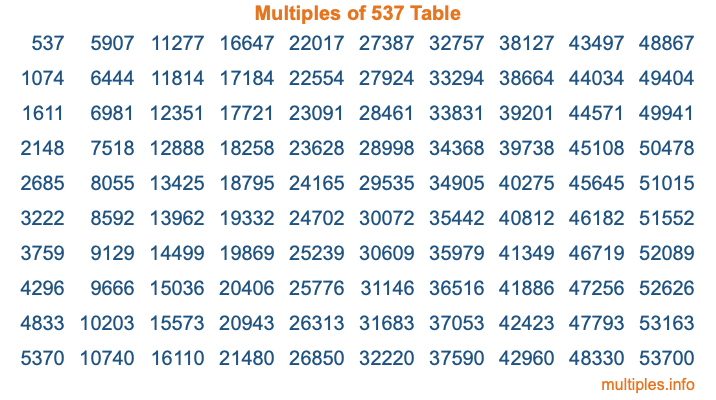Multiples of 537Welcome to the Multiples of 537 page. Here we will first teach you everything you will ever need to know about the multiples of 537, and then give you a study guide summary of everything we taught you to make sure you remember it all. Use this page to look up facts and learn information about the multiples of 537. This page will make you a multiples of five hundred thirty-seven expert!

Definition of Multiples of 537
Multiples of 537 are all the numbers that when divided by 537 equal an integer. Each of the multiples of 537 are called a multiple. A multiple of 537 is created by multiplying 537 by an integer.

Therefore, to create a list of multiples of 537, you start with 1 multiplied by 537, then 2 multiplied by 537, then 3 multiplied by 537, and so on for as long as you want. Thus, the list of the first five multiples of 537 is 537, 1074, 1611, 2148, and 2685. To see a larger list of multiples of 537, see the printable image of Multiples of 537 further down on this page. We also have a category where you can choose any nth multiple of 537.

Multiples of 537 Checker
The Multiples of 537 Checker below checks to see if any number of your choice is a multiple of 537. In other words, it checks to see if there is any number (integer) that when multiplied by 537 will equal your number. To do that, we divide your number by 537. If the the quotient is an integer, then your number is a multiple of 537.

Is  a multiple of 537?

Least Common Multiple of 537 and ...
A Least Common Multiple (LCM) is the lowest multiple that two or more numbers have in common. This is also called the smallest common multiple or lowest common multiple and is useful to know when you are adding our subtracting fractions. Enter one or more numbers below (537 is already entered) to find the LCM.

Check out our LCM Calculator if you need more details about the Least Common Multiple or if you need the LCM for different numbers for adding and subtraction fractions.

nth Multiple of 537
As we stated above, 537 is the first multiple of 537, 1074 is the second multiple of 537, 1611 is the third multiple of 537, and so on. Enter a number below to find the nth multiple of 537.

th multiple of 537

Multiples of 537 vs Factors of 537
537 is a multiple of 537 and a factor of 537, but that is where the similarities end. All postive multiples of 537 are 537 or greater than 537. All positive factors of 537 are 537 or less than 537.

Below is the beginning list of multiples of 537 and the factors of 537 so you can compare:

Multiples of 537: 537, 1074, 1611, 2148, 2685, etc.

Factors of 537: 1, 3, 179, 537

As you can see, the multiples of 537 are all the numbers that you can divide by 537 to get a whole number. The factors of 537, on the other hand, are all the whole numbers that you can multiply by another whole number to get 537.

It's also interesting to note that if a number (x) is a factor of 537, then 537 will also be a multiple of that number (x).

Multiples of 537 vs Divisors of 537
The divisors of 537 are all the integers that 537 can be divided by evenly. Below is a list of the divisors of 537.

Divisors of 537: 1, 3, 179, 537

The interesting thing to note here is that if you take any multiple of 537 and divide it by a divisor of 537, you will see that the quotient is an integer.

Multiples of 537 Table
Below is an image of the first 100 multiples of 537 in a table. The table is in chronological order, column by column. The first column has the first ten multiples of 537, the second column has the next ten multiples of 537, and so on.The Multiples of 537 Table is also referred to as the 537 Times Table or Times Table of 537. You are welcome to print out our table for your studies.

Negative Multiples of 537
Although not often discussed or needed in math, it is worth mentioning that you can make a list of negative multiples of 537 by multiplying 537 by -1, then by -2, then by -3, and so on, to get the following list of negative multiples of 537:

-537, -1074, -1611, -2148, -2685, etc.

Multiples of 537 Summary
Below is a summary of important Multiples of 537 facts that we have discussed on this page. To retain the knowledge on this page, we recommend that you read through the summary and explain to yourself or a study partner why they hold true.

There are an infinite number of multiples of 537.

A multiple of 537 divided by 537 will equal a whole number.

537 divided by a factor of 537 equals a divisor of 537.

The nth multiple of 537 is n times 537.

The largest factor of 537 is equal to the first positive multiple of 537.

537 is a multiple of every factor of 537.

537 is a multiple of 537.

A multiple of 537 divided by a divisor of 537 equals an integer.

537 divided by a divisor of 537 equals a factor of 537.

Any integer times 537 will equal a multiple of 537.

Multiples of a Number
Here you can get the multiples of another number, all with the same attention to detail as we did for multiples of 537 on this page.

Multiples of
Multiples of 538
Did you find our page about multiples of five hundred thirty-seven educational? Do you want more knowledge? Check out the multiples of the next number on our list!